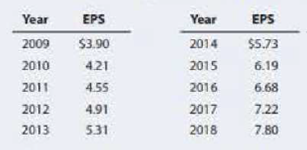Chapter 10, Problem 20PFundamentals of Financial Manageme...

15th Edition
Eugene F. Brigham + 1 other
ISBN: 9781337395250

Solutions

Chapter
SectionFundamentals of Financial Manageme...

15th Edition
Eugene F. Brigham + 1 other
ISBN: 9781337395250
Textbook Problem

WACC The following table gives Foust Company's earnings per share for the last 10 years. The common stock, 7.8 million shares outstanding, is now (1/1/19) selling for \$65.00 per share. The expected dividend at the end of the current year (12/31/19) is 55% of the 2018 EPS. Because investors expect past trends to continue, g may be based on the historical earnings growth rate. (Note that 9 years of growth are reflected in the 10 years of data.)Tire current interest rate on new debt is 9%; Foust's marginal tax rate is 40%, and its target capital structure is 40% debt and 60% equity. a. Calculate Foust's after-tax cost of debt and common equity. Calculate the cost of equity as rs = D1/Pa + g. b. Find Foust's WACC.

a.

Summary Introduction

To Determine: The after-tax cost of debt, cost common equity and cost of equity of Company F.

Introduction: WACC is abbreviated as weighted average cost of capital, is a equation that computes the average rate of return that an organization requires to acquire to repay its security holders or investors. This computation is utilized to determine if a project is beneficial or in the event that it just repays the expense of subsidizing the project.

Explanation

Determine the after-tax cost of debt

AftertaxCostofDebt=[CostofDebt(rd)×(1TaxRate(T))]=[9%×(140%)]=[9%×60%]=5.40%

Therefore the after-tax cost of debt is 5.40%.

Determine the cost of common equity

Using a excel spreadsheet and excel function =RATE, the cost of common equity is determined as 8.01%. There are 9 years from 2009 to 2018.

Therefore the cost of common equity is 8.01%

b.

Summary Introduction

To Determine: The WACC.

Still sussing out bartleby?

Check out a sample textbook solution.

See a sample solution

The Solution to Your Study Problems

Bartleby provides explanations to thousands of textbook problems written by our experts, many with advanced degrees!

Get Started

Find more solutions based on key concepts